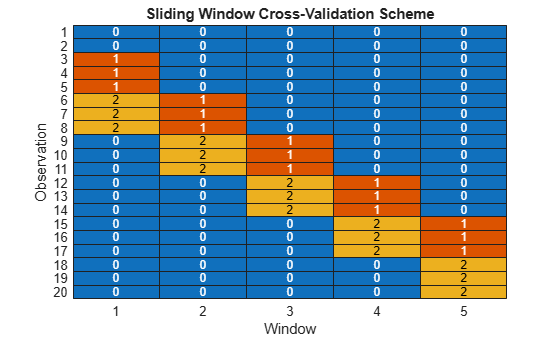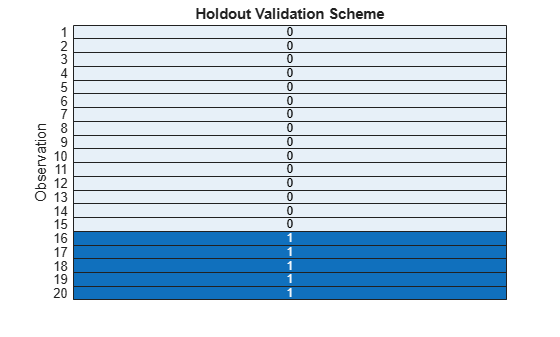# tspartition

Partition time series data for cross-validation

## Description

A `tspartition` object partitions a set of regularly sampled, time series data based on the specified size of the data set. Use this object to define training and test sets for validating a time series regression model with expanding window cross-validation, sliding window cross-validation, or holdout validation. Use the `training` object function to extract the training indices and the `test` object function to extract the test indices.

For an example that uses `tspartition` for time series forecasting, see Time Series Forecasting Using Ensemble of Boosted Regression Trees.

## Creation

### Syntax

``c = tspartition(n,"ExpandingWindow",t)``
``c = tspartition(n,"SlidingWindow",t)``
``c = tspartition(n,"Holdout",p)``
``c = tspartition(___,Name=Value)``

### Description

example

````c = tspartition(n,"ExpandingWindow",t)` creates a `tspartition` object `c` that partitions `n` time-dependent observations using expanding windows. `tspartition` splits the data set into `t` windows with expanding training sets and fixed-size test sets.```

example

````c = tspartition(n,"SlidingWindow",t)` creates a `tspartition` object `c` that partitions `n` time-dependent observations using sliding windows. `tspartition` splits the data set into `t` windows with fixed-size training and test sets.```

example

````c = tspartition(n,"Holdout",p)` creates a `tspartition` object `c` that defines a time-based partition for holdout validation on `n` observations. `tspartition` divides the `n` observations into a training set and a test set, where `p` determines the fraction or number of observations in the test set.```

example

````c = tspartition(___,Name=Value)` specifies options using one or more name-value arguments in addition to any of the input argument combinations in previous syntaxes. For example, you can specify the number of observations to exclude between the end of each training set and before the beginning of its corresponding test set by using the `GapSize` name-value argument.```

### Input Arguments

expand all

Number of observations in the time series data set, specified as a positive integer scalar.

Example: `10000`

Data Types: `single` | `double`

Number of test sets to create, specified as a positive integer scalar. `t` must be smaller than the total number of observations `n`.

Example: `5`

Data Types: `single` | `double`

Fraction or number of observations in the test set used for holdout validation, specified as a scalar in the range (0,1) or a positive integer scalar.

• When `p` is in the range (0,1), `tspartition` selects approximately `p*n` of the latest observations for the test set.

• When `p` is a positive integer, `tspartition` selects the `p` latest observations for the test set.

Data Types: `single` | `double`

Name-Value Arguments

Specify optional pairs of arguments as `Name1=Value1,...,NameN=ValueN`, where `Name` is the argument name and `Value` is the corresponding value. Name-value arguments must appear after other arguments, but the order of the pairs does not matter.

Example: `tspartition(10000,"ExpandingWindow",5,MaxTrainSize=7500)` specifies to split 10,000 observations into 5 partitions with expanding training sets and fixed-size test sets. Each training set cannot contain more than 7500 observations.

Start direction for creating time windows, specified as `"forward"` or `"reverse"`.

• `"forward"``tspartition` ensures that the oldest observations are included in the first window. Some of the latest observations might be omitted from the cross-validation.

• `"reverse"``tspartition` ensures that the latest observations are included in the last window. Some older observations might be omitted from the cross-validation.

Note

This name-value argument is valid for expanding window and sliding window cross-validation only.

Example: `Direction="forward"`

Data Types: `char` | `string`

Number of observations to exclude between the end of each training set and before the beginning of its corresponding test set, specified as a scalar in the range [0,1) or a positive integer scalar.

• When the `GapSize` value is in the range [0,1), `tspartition` excludes approximately `GapSize*n` observations.

• When the `GapSize` value is a positive integer, `tspartition` excludes `GapSize` observations.

Example: `GapSize=10`

Data Types: `single` | `double`

Maximum size of all training sets, specified as a scalar in the range (0,1) or a positive integer scalar.

• When the `MaxTrainSize` value is in the range (0,1), `tspartition` includes at most `MaxTrainSize*n` observations in each training set.

• When the `MaxTrainSize` value is a positive integer, `tspartition` includes at most `MaxTrainSize` observations in each training set.

Note

This name-value argument is valid for expanding window cross-validation only.

Example: `MaxTrainSize=500`

Data Types: `single` | `double`

Minimum size of all training sets, specified as a scalar in the range (0,1) or a positive integer scalar.

• When the `MinTrainSize` value is in the range (0,1), `tspartition` includes at least `MinTrainSize*n` observations in each training set.

• When the `MinTrainSize` value is a positive integer, `tspartition` includes at least `MinTrainSize` observations in each training set.

If you do not specify other name-value arguments, the default value is `floor(n/(t+1))` (see `n` and `t`).

Note

This name-value argument is valid for expanding window cross-validation only.

Example: `MinTrainSize=100`

Data Types: `single` | `double`

Step length between consecutive windows, specified as a scalar in the range (0,1) or a positive integer scalar. More specifically, the `StepSize` value is the number of steps between the end of two consecutive test sets.

• When the `StepSize` value is in the range (0,1), `tspartition` separates consecutive test sets by approximately `StepSize*n` steps.

• When the `StepSize` value is a positive integer, `tspartition` separates consecutive test sets by `StepSize` steps.

If you do not specify other name-value arguments, the default value is `floor(n/(t+1))` (see `n` and `t`).

Note

This name-value argument is valid for expanding window and sliding window cross-validation only.

Example: `StepSize=50`

Data Types: `single` | `double`

Size of all training sets, specified as a scalar in the range (0,1) or a positive integer scalar.

• When the `TrainSize` value is in the range (0,1), `tspartition` includes approximately `TrainSize*n` observations in each training set.

• When the `TrainSize` value is a positive integer, `tspartition` includes `TrainSize` observations in each training set.

If you do not specify other name-value arguments, the default value is `floor(n/(t+1))` (see `n` and `t`).

Note

This name-value argument is valid for sliding window cross-validation only.

Example: `TrainSize=500`

Data Types: `single` | `double`

Size of all test sets, specified as a scalar in the range (0,1) or a positive integer scalar.

• When the `TestSize` value is in the range (0,1), `tspartition` includes approximately `TestSize*n` observations in each test set.

• When the `TestSize` value is a positive integer, `tspartition` includes `TestSize` observations in each test set.

If you do not specify other name-value arguments, the default value is `floor(n/(t+1))` (see `n` and `t`).

Note

This name-value argument is valid for expanding window and sliding window cross-validation only.

Example: `TestSize=100`

Data Types: `single` | `double`

## Properties

expand all

Validation partition type, returned as `'expanding-window'`, `'holdout'`, or `'sliding-window'`.

Data Types: `char`

Number of observations, returned as a positive integer scalar.

Data Types: `single` | `double`

Number of test sets, returned as a positive integer scalar. For holdout validation, the `NumTestSets` value is `1`. For expanding window and sliding window cross-validation, the `NumTestSets` value indicates the number of windows used for cross-validation.

Data Types: `single` | `double`

Size of each training set, returned as a positive integer scalar for holdout validation or a positive integer vector for expanding window and sliding window cross-validation.

Data Types: `single` | `double`

Size of each test set, returned as a positive integer scalar for holdout validation or a positive integer vector for expanding window and sliding window cross-validation.

Data Types: `single` | `double`

Step length between consecutive windows, returned as a positive integer scalar when the `NumTestSets` value is greater than `1`, or `NaN` otherwise.

Data Types: `single` | `double`

## Object Functions

 `test` Test indices for time series cross-validation `training` Training indices for time series cross-validation

## Examples

collapse all

Identify the observations in the training sets and test sets of a `tspartition` object for expanding window cross-validation.

Use 20 time-dependent observations to create three training sets and three test sets. Specify a gap of two observations between each training set and its corresponding test set.

```c = tspartition(20,"ExpandingWindow",3, ... GapSize=2);```

Find the training set indices for the three windows. A value of 1 (`true`) indicates that the corresponding observation is in the training set for that window.

```trainWindow1 = training(c,1); trainWindow2 = training(c,2); trainWindow3 = training(c,3);```

Find the test set indices for the three windows. A value of 1 (`true`) indicates that the corresponding observation is in the test set for that window.

```testWindow1 = test(c,1); testWindow2 = test(c,2); testWindow3 = test(c,3);```

Combine the training and test set indices into one matrix where a value of 1 indicates a training observation and a value of 2 indicates a test observation.

```data = [trainWindow1 + 2*testWindow1, ... trainWindow2 + 2*testWindow2, ... trainWindow3 + 2*testWindow3];```

Visualize the different sets by using a heat map.

```colormap = lines(3); heatmap(double(data),ColorbarVisible="off", ... Colormap=colormap); xlabel("Window") ylabel("Observation") title("Expanding Window Cross-Validation Scheme")```For each window, the observations in red (with a value of 1) are in the training set, the observations in yellow (with a value of 2) are in the test set, and the observations in blue (with a value of 0) are ignored. For example, observation 11 is a test observation in window one, a gap observation in window two, and a training observation in window three.

Identify the observations in the training sets and test sets of a `tspartition` object for sliding window cross-validation.

Use 20 time-dependent observations to create five training sets and five test sets.

`c = tspartition(20,"SlidingWindow",5);`

Find the training set indices for the five windows. A value of 1 (`true`) indicates that the corresponding observation is in the training set for that window.

```trainWindows = zeros(c.NumObservations,c.NumTestSets); for i = 1:c.NumTestSets trainWindows(:,i) = training(c,i); end```

Find the test set indices for the five windows. A value of 1 (`true`) indicates that the corresponding observation is in the test set for that window.

```testWindows = zeros(c.NumObservations,c.NumTestSets); for i = 1:c.NumTestSets testWindows(:,i) = test(c,i); end```

Combine the training and test set indices into one matrix where a value of 1 indicates a training observation and a value of 2 indicates a test observation.

`data = trainWindows + 2*testWindows;`

Visualize the different sets by using a heat map.

```colormap = lines(3); heatmap(double(data),ColorbarVisible="off", ... Colormap=colormap); xlabel("Window") ylabel("Observation") title("Sliding Window Cross-Validation Scheme")```For each window, the observations in red (with a value of 1) are in the training set, the observations in yellow (with a value of 2) are in the test set, and the observations in blue (with a value of 0) are ignored. For example, observations 9 through 11 are test observations in window two and training observations in window three. Because of the default values for the training set size, test set size, step size, and direction for creating sliding windows, `tspartition` does not use some of the oldest observations (1 and 2) in any window.

Identify the observations in the training set and test set of a `tspartition` object for holdout validation.

Use 25% of 20 time-dependent observations to create a test set. The corresponding training set contains the remaining observations.

`c = tspartition(20,"Holdout",0.25);`

Find the test set indices.

`testIndices = test(c);`

Visualize the two sets of observations by using a heat map.

```h = heatmap(double(testIndices),ColorbarVisible="off"); h.XDisplayLabels = ""; ylabel("Observation") title("Holdout Validation Scheme")```The observations in light blue (with a value of 0) are in the training set, and the observations in dark blue (with a value of 1) are in the test set. In a holdout validation scheme for time series data, the latest observations (in this case, observations 16 through 20) are in the test set.

## Version History

Introduced in R2022b